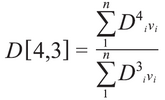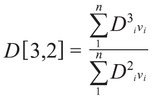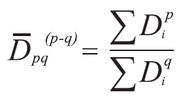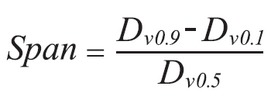Scientific

# Understanding and Interpreting Particle Size Distribution Calculations

Performing a particle size analysis is the best way to answer the question: What size are those particles? Once the analysis is complete the user has a variety of approaches for reporting the result. Some people prefer a single number answer—what is the average size? More experienced particle scientists cringe when they hear this question, knowing that a single number cannot describe the distribution of the sample. A better approach is to report both a central point of the distribution along with one or more values to describe the width of distribution. Other approaches are also described in this web page.

## Central Values: Mean, Median, and Mode

For symmetric distributions such as the one shown in Figure 1 all central values are equivalent: mean = median = mode. But what do these values represent?

### Mean

Mean is a calculated value similar to the concept of average. The various mean calculations are defined in several standard documents (ref.1,2). There are multiple definitions for mean because the mean value is associated with the basis of the distribution calculation (number, surface, volume). See (ref. 3) for an explanation of number, surface, and volume distributions. Laser diffraction results are reported on a volume basis, so the volume mean can be used to define the central point although the median is more frequently used than the mean when using this technique. The equation for defining the volume mean is shown below. The best way to think about this calculation is to think of a histogram table showing the upper and lower limits of n size channels along with the percent within this channel. The Di value for each channel is the geometric mean, the square root of upper x lower diameters. For the numerator take the geometric Di to the fourth power multiplied by the percent in that channel, summed over all channels. For the denominator take the geometric Di to the third power multiplied by the percent in that channel, summed over all channels.The volume mean diameter has several names including D4,3. In all HORIBA diffraction software this is simply called the “mean” whenever the result is displayed as a volume distribution. Conversely, when the result in HORIBA software is converted to a surface area distribution the mean value displayed is the surface mean, or D3,2. The equation for the surface mean is shown below.The description for this calculation is the same as the D4,3 calculation, except that Di values are raised to the exponent values of 3 and 2 instead of 4 and 3. The generalized form of the equations seen above for D4,3 and D3,2 is shown below (following the conventions from ref. 2, ASTM E 799).Where:

• D‾            = the overbar in D designates an averaging process
• (p-q)p>q  = the algebraic power of Dpq
• Di             = the diameter of the ith particle
• Σ              = the summation of Dip or Diq, representing all particles in the sample

Some of the more common representative diameters are:

• D‾10          = arithmetic or number mean
• D‾32          = volume/surface mean (also called the Sauter mean)
• D‾43          = the mean diameter over volume (also called the de Brouckere mean)

The example results shown in ASTM E 799 are based on a distribution of liquid droplets (particles) ranging from 240 - 6532 µm. For this distribution the following results were calculated:

• D(1,0)         = 1460 µm
• D(3,2)         = 2280 µm
• D50            = 2540 µm
• D(4,3)         = 2670 µm

These results are fairly typical in that the D(4,3) is larger than the D50 - the volume-basis median value.

### Median

Median values are defined as the value where half of the population resides above this point, and half resides below this point. For particle size distributions the median is called the D50 (or x50 when following certain ISO guidelines). The D50 is the size in microns that splits the distribution with half above and half below this diameter. The Dv50 (or Dv0.5) is the median for a volume distribution, Dn50 is used for number distributions, and Ds50 is used for surface distributions. Since the primary result from laser diffraction is a volume distribution, the default D50 cited is the volume median and D50 typically refers to the Dv50 without including the v. This value is one of the easier statistics to understand and also one of the most meaningful for particle size distributions.

### Mode

The mode is the peak of the frequency distribution, or it may be easier to visualize it as the highest peak seen in the distribution. The mode represents the particle size (or size range) most commonly found in the distribution. Less care is taken to denote whether the value is based on volume, surface or number, so either run the risk of assuming volume basis or check to assure the distribution basis. The mode is not as commonly used, but can be descriptive; in particular if there is more than one peak to the distribution, then the modes are helpful to describe the mid-point of the different peaks.

For non-symmetric distributions the mean, median and mode will be three different values shown in Figure 2.

## Distribution Widths

Most instruments are used to measure the particle size distribution, implying an interest in the width or breadth of the distribution. Experienced scientists typically shun using a single number answer to the question “What size are those particles?”, and prefer to include a way to define the width. The field of statistics provides several calculations to describe the width of distributions, and these calculations are sometimes used in the field of particle characterization. The most common calculations are standard deviation and variance. The standard deviation (St Dev.) is the preferred value in our field of study. As shown in Figure 3, 68.27% of the total population lies within +/- 1 St Dev, and 95.45% lies within +/- 2 St Dev. Although occasionally cited, the use of standard deviation declined when hardware and software advanced beyond assuming normal or Rosin-Rammler distributions. Once “model independent” algorithms were introduced many particle scientists began using different calculations to describe distribution width. One of the common values used for laser diffraction results is the span, with the strict definition shown in the equation below (2):In rare situations the span equation may be defined using other values such as Dv0.8 and Dv0.2. Laser diffraction instruments should allow users this flexibility.

An additional approach to describing distribution width is to normalize the standard deviation through division by the mean. This is the Coefficient of Variation (COV) (although it may also be referred to as the relative standard deviation, or RSD). Although included in HORIBA laser diffraction software this value is seldom used as often as it should given its stature. The COV calculation is both used and encouraged as a calculation to express measurement result reproducibility. ISO 13320 (ref. 4) encourages all users to measure any sample at least 3 times, calculate the mean, st dev, and COV (st dev/mean), and the standard sets pass/fail criteria based on the COV values.

Another common approach to define the distribution width is to cite three values on the x-axis, the D10, D50, and D90 as shown in Figure 4. The D50, the median, has been defined above as the diameter where half of the population lies below this value. Similarly, 90 percent of the distribution lies below the D90, and 10 percent of the population lies below the D10.

## Technique Dependence

HORIBA Scientific offers particle characterization tools based on several principles including laser diffraction, dynamic light scattering, and image analysis. Each of these techniques generates results in both similar and unique ways. Most techniques can describe results using standard statistical calculations such as the mean and standard deviation. But commonly accepted practices for describing results have evolved for each technique.

### Laser Diffraction

All of the calculations described in this document are generated by the HORIBA laser diffraction software package. Results can be displayed on a volume, surface area, or number basis. Statistical calculations such as standard deviation and variance are available in either arithmetic or geometric forms. The most common approach for expressing laser diffraction results is to report the D10, D50, and D90 values based on a volume distribution. The span calculation is the most common format to express distribution width. That said, there is nothing wrong with using any of the available calculations, and indeed many customers include the D4,3 when reporting results.

A word of caution is given when considering converting a volume distribution into either a surface area or number basis. Although the conversion is supplied in the software, it is only provided for comparison to other techniques, such as microscopy, which inherently measure particles on different bases. The conversion is only valid for symmetric distributions and should not be used for any other purpose than comparison to another technique.

### Dynamic Light Scattering

Dynamic Light Scattering (DLS) is unique among the techniques described in this document. The primary result from DLS is typically the intensity based harmonic mean value and is typically called the Z-average. (See the What is the Z-Average? web page for more information). Distribution width is described using the polydispersity index (PDI). It is possible to convert from an intensity to a volume or number distribution in order to compare to other techniques.

### Image Analysis

The primary results from image analysis are based on number distributions. These are often converted to a volume basis, and in this case this is an accepted and valid conversion. Image analysis provides far more data values and options than any of the other techniques described in this document. Measuring each particle allows the user unmatched flexibility for calculating and reporting particle size results.

Image analysis instruments may report distributions based on particle length as opposed to spherical equivalency, and they may build volume distributions based on shapes other than spheres.

Image analysis tools allow users to choose a variety of length and width descriptors such as the maximum Feret diameter and the minimum largest chord diameter as described in ISO 13322-2 (ref. 5).

With the ability to measure particles in any number of ways comes the decision to report those measurements in any number of ways. Users are again cautioned against reporting a single value—the number mean being the worst choice of the possible options. Experienced particle scientists often report D10, D50, and D90, or include standard deviation or span calculations when using image analysis tools.

## Conclusions

All particle size analysis instruments provide the ability to measure and report the particle size distribution of the sample. There are very few applications where a single value is appropriate and representative. The modern particle scientist often chooses to describe the entire size distribution as opposed to just a single point on it. (One exception might be extremely narrow distributions such as latex size standards where the width is negligible.) Almost all real world samples exist as a distribution of particle sizes and it is recommended to report the width of the distribution for any sample analyzed. The most appropriate option for expressing width is dependent on the technique used. When in doubt, it is often wise to refer to industry accepted standards such as ISO or ASTM in order to conform to common practice.

## References

1. ISO 9276-2:2001 : Representation of results of particle size analysis – Part 2: Calculation of average particle sizes/diameters and moments from particle size distributions
2. ASTM E 799-03 Standard Practice for Determining Data Criteria and Processing for Liquid Drop Size Analysis
3. TN154, Particle Size Result Interpretation: Number vs. Volume Distributions, available here.
4. ISO 13320-1 Particle size analysis – Laser diffraction methods
5. ISO 13322-2 Particle size analysis – Image analysis methods – Part 2: Dynamic image analysis methods

## Browse Products

Partica LA-960V2

Laser Scattering Particle Size Distribution Analyzer

Partica mini LA-350

Laser Scattering Particle Size Distribution Analyzer

ViewSizer 3000

Multi-Laser Nanoparticle Tracking Analysis (NTA)

nanoPartica SZ-100V2 Series

Nanoparticle Analyzer

Eyecon2™*

Direct Imaging Particle Analyzer

PSA300*

Static Image Analysis System Particle Size

SA-9600 Series

BET Flowing Gas Surface Area Analyzers

### REQUEST FOR INFORMATION

Do you have any questions or requests? Use this form to contact our specialists.